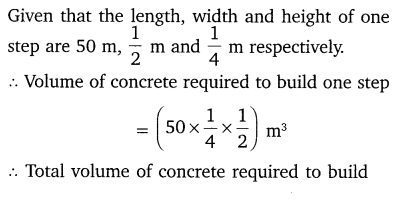# CLASS 10 MATH NCERT SOLUTIONS FOR CHAPTER – 5 ARITHMETIC PROGRESSIONS EX – 5.4

## Arithmetic Progressions

Question 1.
Which term of the AP: 121, 117. 113, ….., is its first negative term?

Solution: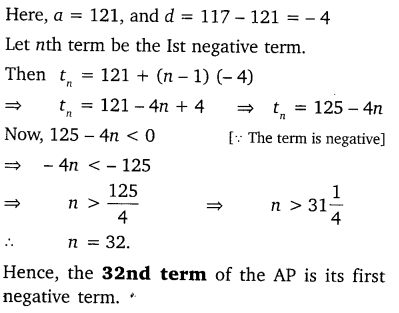Question 2.
The sum of the third term and the seventh term of an AP is 6 and their product is 8. Fibd the sum of first sixteen terms of the AP?

Solution: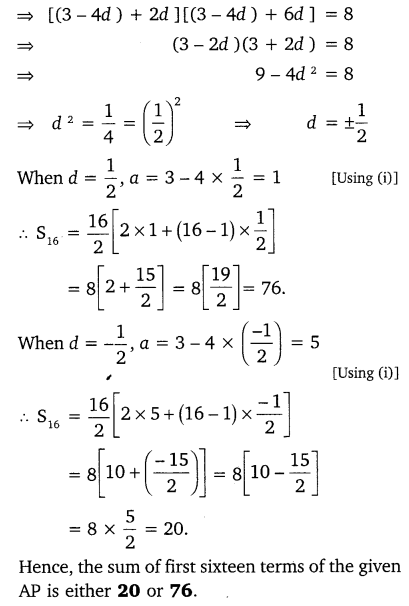Question 3.
A ladder has rungs 25 cm apart. The rungs decrease uniformly in length from 45 cm at the bottom to 25 at the top. If the top and the bottom rungs are${ 2 }\frac { 1 }{ 2 } m$ apart, what is the length of the wood required for the rungs?

Solution: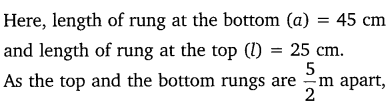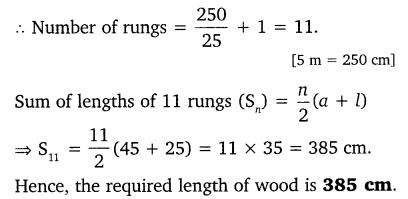Question 4.
The houses of a row are numbered consecutively from 1 to 49. Show that there is value of x such that the sum of the numbers of the houses preceding the house numbered x is equal to the sum of the numbers of the houses following it. Find this value of x.

Solution:Question 5.
A small terrace at a football ground comprises of 15 steps each of which is 50  m long and built of solid concrete. Each step has a rise of$\frac { 1 }{ 2 } m$ and a tread of$\frac { 1 }{ 2 } m$. Calculate the total volume of concrete required to build the terrace.

Solution: